# TensorFlow笔记6：MNIST 2

## Jan 15,2018   14090 words   51 min

Tags: DeepLearning

### 一、模型训练代码

``````# coding= utf-8
import tensorflow as tf
import time

def weight_variable(shape, Name=None):
# 截断正态分布（在-2u - 2u之间），更多可看函数的documentation
# 这个函数用于返回一个指定shape的tensor，其中的数值符合正态分布
# 模型中的权重在初始化时应该加入少量的噪声来打破对称性以及避免0梯度，因此标准差设为0.1
# 注意这里的标准差初始值不能设置的太大，如2，否则会出现精度往复，无法提升的情况
initial = tf.truncated_normal(shape, stddev=0.1)
# 表示用初值创建一个TF变量并返回
return tf.Variable(initial, name=Name)

def bias_variable(shape, Name=None):
# 创建一个指定shape的常量tensor
# 由于我们使用的是ReLU神经元，因此比较好的做法是用一个较小的正数来初始化偏置项
initial = tf.constant(0.1, shape=shape)
return tf.Variable(initial, name=Name)

def conv2d(x, W):
# 卷积函数，x和W分别为输入图像和卷积核
return tf.nn.conv2d(x, W, strides=[1, 1, 1, 1], padding="SAME")

def max_pool_2x2(x):
# 池化函数，表示选择2×2共4个像素中的最大值作为合并后的新像素值
return tf.nn.max_pool(x, ksize=[1, 2, 2, 1], strides=[1, 2, 2, 1], padding="SAME")

count = int(input("Specify train times:\n"))
nums = int(input("Specify number of images for every batch:\n"))
print("training...")

# 1.载入数据
from tensorflow.examples.tutorials.mnist import input_data

# 这里的x和y_分别表示每一张输入图像和其对应的标签
x = tf.placeholder(tf.float32, [None, 784])
y_ = tf.placeholder(tf.float32, [None, 10])
# 将输入的x向量变成28×28的图像
x_images = tf.reshape(x, [-1, 28, 28, 1])

# 第一层卷积
# 卷积大小为5×5，初始值随机且符合正态分布
# 输入通道数为1，卷积个数为32
W_conv1 = weight_variable([5, 5, 1, 32], Name='W_conv1')
b_conv1 = bias_variable(, Name="b_conv1")
# 使用ReLU激活函数
h_conv1 = tf.nn.relu(conv2d(x_images, W_conv1) + b_conv1)
h_pool1 = max_pool_2x2(h_conv1)

# 第二层卷积
W_conv2 = weight_variable([5, 5, 32, 64], Name='W_conv2')
b_conv2 = bias_variable(, Name="b_conv2")

h_conv2 = tf.nn.relu(conv2d(h_pool1, W_conv2) + b_conv2)
h_pool2 = max_pool_2x2(h_conv2)

# 全连接层
# 经过两层卷积，现在图像大小是7×7，且“厚度”为64
# 可以理解为对于第一层卷积后得到的14×14大小的图像用64个不同的卷积核进行卷积
# 最后得到64个7×7大小的卷积后的结果图像
# 而1024是人为指定的，表示全连接层有1024个神经元
# 全连接层的作用是把卷积输出的二维特征图转化成一维的一个向量
# 其实可以理解为有64*1024个7*7的卷积核卷积出来的
# 对于输入的每一张图，用了一个和图像一样大小的核卷积，这样整幅图就变成了一个数了
# 如果厚度是64就是那64个核卷积完了之后相加求和。这样就能把一张图高度浓缩成一个数了。
W_fc1 = weight_variable([7 * 7 * 64, 1024])
b_fc1 = bias_variable()

# 下一步要进行矩阵相乘，因此这里reshape一下输入
# 可以理解为把所有卷积的结果全部变成一维线性的
h_pool2_flat = tf.reshape(h_pool2, [-1, 7 * 7 * 64])
h_fc1 = tf.nn.relu(tf.matmul(h_pool2_flat, W_fc1) + b_fc1)

# Dropout，防止过拟合
# Dropout就是在不同的训练过程中随机扔掉一部分神经元。
# 也就是让某个神经元的激活值以一定的概率p，让其停止工作，这次训练过程中不更新权值，也不参加神经网络的计算。
# 但是它的权重得保留下来（只是暂时不更新而已），因为下次样本输入时它可能又得工作了。
keep_prob = tf.placeholder(tf.float32)
h_fc1_drop = tf.nn.dropout(h_fc1, keep_prob)

# 输出层
W_fc2 = weight_variable([1024, 10])
b_fc2 = bias_variable()
y_conv = tf.nn.softmax(tf.matmul(h_fc1_drop, W_fc2) + b_fc2)

# 评价指标、模型的构建
cross_entropy = -tf.reduce_sum(y_ * tf.log(y_conv))
correct_prediction = tf.equal(tf.argmax(y_conv, 1), tf.argmax(y_, 1))
accuracy = tf.reduce_mean(tf.cast(correct_prediction, tf.float32))
saver = tf.train.Saver()

# 训练
# 写代码的时候，有时候会忘记写sess.close().我们可以使用系统的带的with来实现session的自动关闭
start = time.time()
with tf.Session() as sess:
init = tf.global_variables_initializer()
sess.run(init)

for i in range(count):
batch = mnist.train.next_batch(nums)
if i % 100 == 0:
print("step", i, "accuracy", sess.run(accuracy, feed_dict={x: batch, y_: batch, keep_prob: 1.0}))
sess.run(train_step, feed_dict={x: batch, y_: batch, keep_prob: 0.5})

test_batch = mnist.train.next_batch(100)
print("random test accuracy", sess.run(accuracy, feed_dict={x: test_batch, y_: test_batch, keep_prob: 1.0}))

# 由于W维数过多，因此无法使用Numpy保存，会报错,因此使用TF的参数保存方法
# 加载文件中的参数数据，会根据name加载数据并保存到变量W和b中
saver.save(sess, './params.ckpt')
print("Parameters are saved.")
end = time.time()
print((end - start), "seconds in total.")
``````

#### 1.截断正态分布

Normal Distribution称为正态分布，也称为高斯分布，Truncated Normal Distribution一般翻译为截断正态分布，也有称为截尾正态分布。

• 限制取值上限，例如，负无穷<x<50
• 限制取值下限，例如，0<x<正无穷
• 上限下限取值都限制，例如，0<x<50

#### 训练结果

##### 1.10000次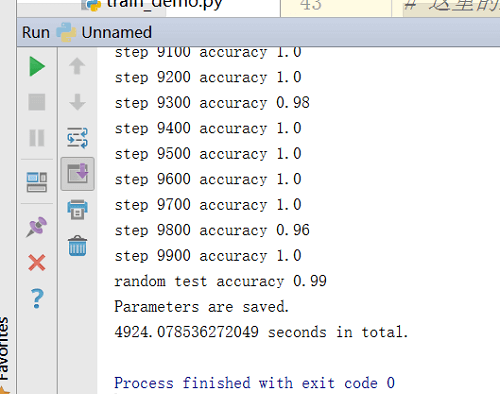• CPU:Intel Celeron N3450
• 平均耗时:49.2s/百步
• 随机测试精度:0.99
##### 2.20000次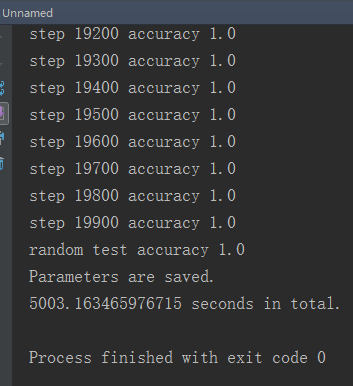• CPU:Intel Core i5 2430M
• 平均耗时:50.03s/百步
• 随机测试精度:1.0
##### 3.30000次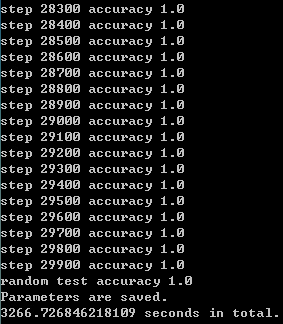• CPU:Intel Xeon X5670
• 平均耗时:10.89s/百步
• 随机测试精度:1.0
##### 4.40000次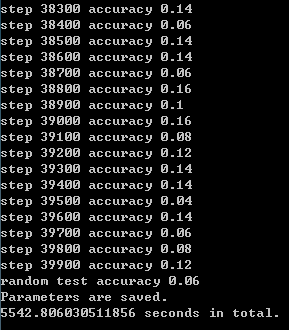• CPU:Intel Xeon X5670
• 平均耗时:13.86s/百步
• 随机测试精度:0.06
##### 5.50000次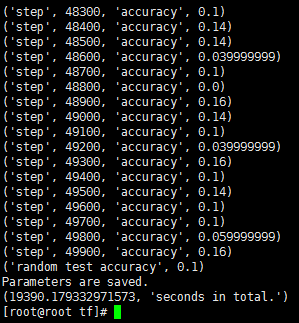• CPU:Intel Xeon E5-2682 v4
• 平均耗时:38.78s/百步
• 随机测试精度:0.1
##### 6.100000次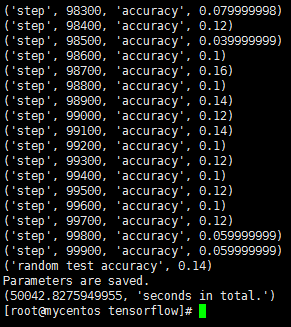• CPU:Intel Xeon E5-2630L v2
• 平均耗时:50.04s/百步
• 随机测试精度:0.14

### 二、模型测试代码

``````# coding= utf-8
import tensorflow as tf

def weight_variable(shape, Name=None):
initial = tf.truncated_normal(shape, stddev=0.1)
return tf.Variable(initial, name=Name)

def bias_variable(shape, Name=None):
initial = tf.constant(0.1, shape=shape)
return tf.Variable(initial, name=Name)

def conv2d(x, W):
return tf.nn.conv2d(x, W, strides=[1, 1, 1, 1], padding="SAME")

def max_pool_2x2(x):
return tf.nn.max_pool(x, ksize=[1, 2, 2, 1], strides=[1, 2, 2, 1], padding="SAME")

count = int(input("Specify test times:\n"))
nums = int(input("Specify number of images for every test:\n"))

# 1.载入数据
from tensorflow.examples.tutorials.mnist import input_data

x = tf.placeholder(tf.float32, [None, 784])
y_ = tf.placeholder(tf.float32, [None, 10])
x_images = tf.reshape(x, [-1, 28, 28, 1])

# 第一层卷积
W_conv1 = weight_variable([5, 5, 1, 32], Name='W_conv1')
b_conv1 = bias_variable(, Name="b_conv1")
h_conv1 = tf.nn.relu(conv2d(x_images, W_conv1) + b_conv1)
h_pool1 = max_pool_2x2(h_conv1)

# 第二层卷积
W_conv2 = weight_variable([5, 5, 32, 64], Name='W_conv2')
b_conv2 = bias_variable(, Name="b_conv2")
h_conv2 = tf.nn.relu(conv2d(h_pool1, W_conv2) + b_conv2)
h_pool2 = max_pool_2x2(h_conv2)

# 全连接层
W_fc1 = weight_variable([7 * 7 * 64, 1024])
b_fc1 = bias_variable()
h_pool2_flat = tf.reshape(h_pool2, [-1, 7 * 7 * 64])
h_fc1 = tf.nn.relu(tf.matmul(h_pool2_flat, W_fc1) + b_fc1)

# 输出层
W_fc2 = weight_variable([1024, 10])
b_fc2 = bias_variable()
y_conv = tf.nn.softmax(tf.matmul(h_fc1, W_fc2) + b_fc2)

# 评价指标、模型的构建
cross_entropy = -tf.reduce_sum(y_ * tf.log(y_conv))
correct_prediction = tf.equal(tf.argmax(y_conv, 1), tf.argmax(y_, 1))
accuracy = tf.reduce_mean(tf.cast(correct_prediction, tf.float32))
saver = tf.train.Saver()

accurs = []

# 测试
with tf.Session() as sess:
init = tf.global_variables_initializer()
sess.run(init)
# 加载文件中的参数数据
saver.restore(sess, './params.ckpt')

for i in range(count):
batch = mnist.train.next_batch(nums)
acc = sess.run(accuracy, feed_dict={x: batch, y_: batch})
accurs.append(acc)
print("test", (i + 1), "accuracy", acc)
print("final average accuracy", sum(accurs) / accurs.__len__())
``````

### 三、更一般的训练、测试代码

#### 1.训练代码

``````# coding= utf-8
import tensorflow as tf
import time

def weight_variable(shape, Name=None):
initial = tf.truncated_normal(shape, stddev=0.1)
return tf.Variable(initial, name=Name)

def bias_variable(shape, Name=None):
initial = tf.constant(0.1, shape=shape)
return tf.Variable(initial, name=Name)

def conv2d(x, W):
return tf.nn.conv2d(x, W, strides=[1, 1, 1, 1], padding="SAME")

def max_pool_2x2(x):
return tf.nn.max_pool(x, ksize=[1, 2, 2, 1], strides=[1, 2, 2, 1], padding="SAME")

# 网络相关参数
# 第一层卷积核个数
num_of_kernel_conv1 = 32
# 第二层卷积核个数
num_of_kernel_conv2 = 64
# 全连接层神经元个数
num_of_neu_fc = 2048

# 指定训练的次数以及每次训练随机抽样的图像个数
count = int(input("Specify train times:\n"))
nums = int(input("Specify number of images for every batch:\n"))
print("training...")

# 载入数据
from tensorflow.examples.tutorials.mnist import input_data

# 这里的x和y_分别表示每一张输入图像和其对应的标签
x = tf.placeholder(tf.float32, [None, 784])
y_ = tf.placeholder(tf.float32, [None, 10])
# 将输入的x向量变成28×28的图像
x_images = tf.reshape(x, [-1, 28, 28, 1])

# 第一层卷积
# 卷积大小为5×5，初始值随机且符合正态分布
# 输入通道数为1，卷积个数为num_of_kernel_conv1
W_conv1 = weight_variable([5, 5, 1, num_of_kernel_conv1], Name='W_conv1')
b_conv1 = bias_variable([num_of_kernel_conv1], Name="b_conv1")
# 使用ReLU激活函数
h_conv1 = tf.nn.relu(conv2d(x_images, W_conv1) + b_conv1)
h_pool1 = max_pool_2x2(h_conv1)

# 第二层卷积
W_conv2 = weight_variable([5, 5, num_of_kernel_conv1, num_of_kernel_conv2], Name='W_conv2')
b_conv2 = bias_variable([num_of_kernel_conv2], Name="b_conv2")

h_conv2 = tf.nn.relu(conv2d(h_pool1, W_conv2) + b_conv2)
h_pool2 = max_pool_2x2(h_conv2)

# 全连接层
# 经过两层卷积，现在图像大小是7×7，且“厚度”为num_of_kernel_conv2
# 可以理解为对于第一层卷积后得到的14×14大小的图像用num_of_kernel_conv2个不同的卷积核进行卷积
W_fc1 = weight_variable([7 * 7 * num_of_kernel_conv2, num_of_neu_fc])
b_fc1 = bias_variable([num_of_neu_fc])

# 下一步要进行矩阵相乘，因此这里reshape一下输入
# 可以理解为把所有卷积的结果全部变成一维线性的
h_pool2_flat = tf.reshape(h_pool2, [-1, 7 * 7 * num_of_kernel_conv2])
h_fc1 = tf.nn.relu(tf.matmul(h_pool2_flat, W_fc1) + b_fc1)

# Dropout，防止过拟合
keep_prob = tf.placeholder(tf.float32)
h_fc1_drop = tf.nn.dropout(h_fc1, keep_prob)

# 输出层
W_fc2 = weight_variable([num_of_neu_fc, 10])
b_fc2 = bias_variable()
y_conv = tf.nn.softmax(tf.matmul(h_fc1_drop, W_fc2) + b_fc2)

# 评价指标、模型的构建
cross_entropy = -tf.reduce_sum(y_ * tf.log(y_conv))
correct_prediction = tf.equal(tf.argmax(y_conv, 1), tf.argmax(y_, 1))
accuracy = tf.reduce_mean(tf.cast(correct_prediction, tf.float32))
saver = tf.train.Saver()

# 训练
# 写代码的时候，有时候会忘记写sess.close().我们可以使用系统的带的with来实现session的自动关闭
start = time.time()
with tf.Session() as sess:
init = tf.global_variables_initializer()
sess.run(init)

for i in range(count):
batch = mnist.train.next_batch(nums)
if i % 100 == 0:
print("step", i, "accuracy", sess.run(accuracy, feed_dict={x: batch, y_: batch, keep_prob: 1.0}))
sess.run(train_step, feed_dict={x: batch, y_: batch, keep_prob: 0.5})

test_batch = mnist.train.next_batch(100)
print("random test accuracy", sess.run(accuracy, feed_dict={x: test_batch, y_: test_batch, keep_prob: 1.0}))

# 由于W维数过多，因此无法使用Numpy保存，会报错,因此使用TF的参数保存方法
# 加载文件中的参数数据，会根据name加载数据并保存到变量W和b中
saver.save(sess, './params.ckpt')
print("Parameters are saved.")
end = time.time()
print((end - start), "seconds in total.")
``````

1024个神经元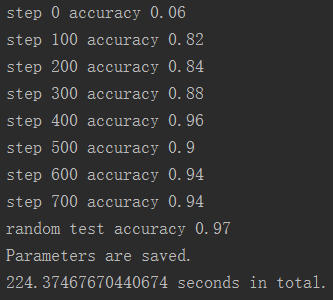2048个神经元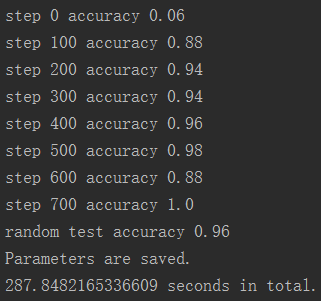可以发现有比较明显的差别，下面的训练精度提升较快。2048大约在200步时精度就到了0.9左右，而1024在400步才到0.9左右。 但尝试将第一层和第二层的卷积核个数翻倍，在有限次的训练下发现精度反而下降了。 可能是因为参数增加了很多，所以训练所需要的次数也增加了，训练更慢了。 但对于这个比较简单的网络，可能并没有必要设置那么多参数，这样不仅精度提升不明显，还会增加计算量，拖慢训练速度。 所以合理选择参数和训练次数对模型的最终结果影响很大。 就像上面说的，训练次数不一定越多越好，同理，参数个数也并不是越多越好。 而是要根据模型的需求和特点，不断调试，找到个较好的平衡点。

#### 2.测试代码

``````# coding=utf-8
import cv2
import tensorflow as tf
import numpy as np
from matplotlib import pyplot as plt

def weight_variable(shape, Name=None):
initial = tf.truncated_normal(shape, stddev=0.1)
return tf.Variable(initial, name=Name)

def bias_variable(shape, Name=None):
initial = tf.constant(0.1, shape=shape)
return tf.Variable(initial, name=Name)

def conv2d(x, W):
return tf.nn.conv2d(x, W, strides=[1, 1, 1, 1], padding="SAME")

def max_pool_2x2(x):
return tf.nn.max_pool(x, ksize=[1, 2, 2, 1], strides=[1, 2, 2, 1], padding="SAME")

# parameters for network
num_of_kernel_conv1 = 32
num_of_kernel_conv2 = 64
num_of_neu_fc = 2048

flag = input("Select mode:\nUse your own image\nUse test dataset\n")
if int(flag) is 1:
path = input("Input image path:\n")
# 读取图片测试，注意图片要求宽高相同
input_image = cv2.resize(input_image, (28, 28), interpolation=cv2.INTER_AREA)
input_image = np.float32(np.dot(input_image, 1.0 / 255.0))
input_image = np.float32(np.abs(input_image - 1.0))
elif int(flag) is 2:
image_index = int(input("Input image index:\n"))
# 读入测试数据集测试
images = mnist.test.images
img = np.reshape(images[image_index], [1, 784])
input_image = img.reshape(28, 28)
else:
print("Invalid input.")

x_image = tf.reshape(input_image, [-1, 28, 28, 1])

# 第一层卷积
W_conv1 = weight_variable([5, 5, 1, num_of_kernel_conv1], Name='W_conv1')
b_conv1 = bias_variable([num_of_kernel_conv1], Name="b_conv1")
h_conv1 = tf.nn.relu(conv2d(x_image, W_conv1) + b_conv1)
h_pool1 = max_pool_2x2(h_conv1)

# 第二层卷积
W_conv2 = weight_variable([5, 5, num_of_kernel_conv1, num_of_kernel_conv2], Name='W_conv2')
b_conv2 = bias_variable([num_of_kernel_conv2], Name="b_conv2")
h_conv2 = tf.nn.relu(conv2d(h_pool1, W_conv2) + b_conv2)
h_pool2 = max_pool_2x2(h_conv2)

# 全连接层
W_fc1 = weight_variable([7 * 7 * num_of_kernel_conv2, num_of_neu_fc])
b_fc1 = bias_variable([num_of_neu_fc])
h_pool2_flat = tf.reshape(h_pool2, [-1, 7 * 7 * num_of_kernel_conv2])
h_fc1 = tf.nn.relu(tf.matmul(h_pool2_flat, W_fc1) + b_fc1)

# 输出层
W_fc2 = weight_variable([num_of_neu_fc, 10])
b_fc2 = bias_variable()
y_conv = tf.nn.softmax(tf.matmul(h_fc1, W_fc2) + b_fc2)

# 评价指标、模型的构建
saver = tf.train.Saver()

# 测试
with tf.Session() as sess:
init = tf.global_variables_initializer()
sess.run(init)
# 加载文件中的参数数据
saver.restore(sess, './params.ckpt')

result = sess.run(y_conv)
final = np.where(result == max(result))
print("final result:", final, "(", max(result), ")")
print("Probabilities:\n", result)

plt.imshow(input_image, cmap="gray")
plt.show()
``````